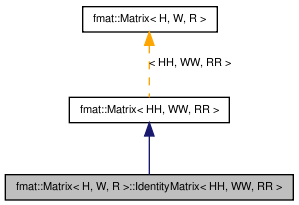# fmat::Matrix< H, W, R >::IdentityMatrix< HH, WW, RR > Struct Template Reference

`#include <fmatCore.h>`

Inheritance diagram for fmat::Matrix< H, W, R >::IdentityMatrix< HH, WW, RR >:[legend]

## Detailed Description

### template<size_t H, size_t W, typename R = fmatReal> template<size_t HH, size_t WW, typename RR = fmatReal> struct fmat::Matrix< H, W, R >::IdentityMatrix< HH, WW, RR >

Definition at line 732 of file fmatCore.h.

List of all members.

## Public Member Functions

IdentityMatrix ()

## Constructor & Destructor Documentation

template<size_t H, size_t W, typename R = fmatReal>
template<size_t HH, size_t WW, typename RR = fmatReal>
 fmat::Matrix< H, W, R >::IdentityMatrix< HH, WW, RR >::IdentityMatrix ( )

Definition at line 733 of file fmatCore.h.

The documentation for this struct was generated from the following file:

 Tekkotsu v5.1CVS Generated Mon May 9 04:59:22 2016 by Doxygen 1.6.3